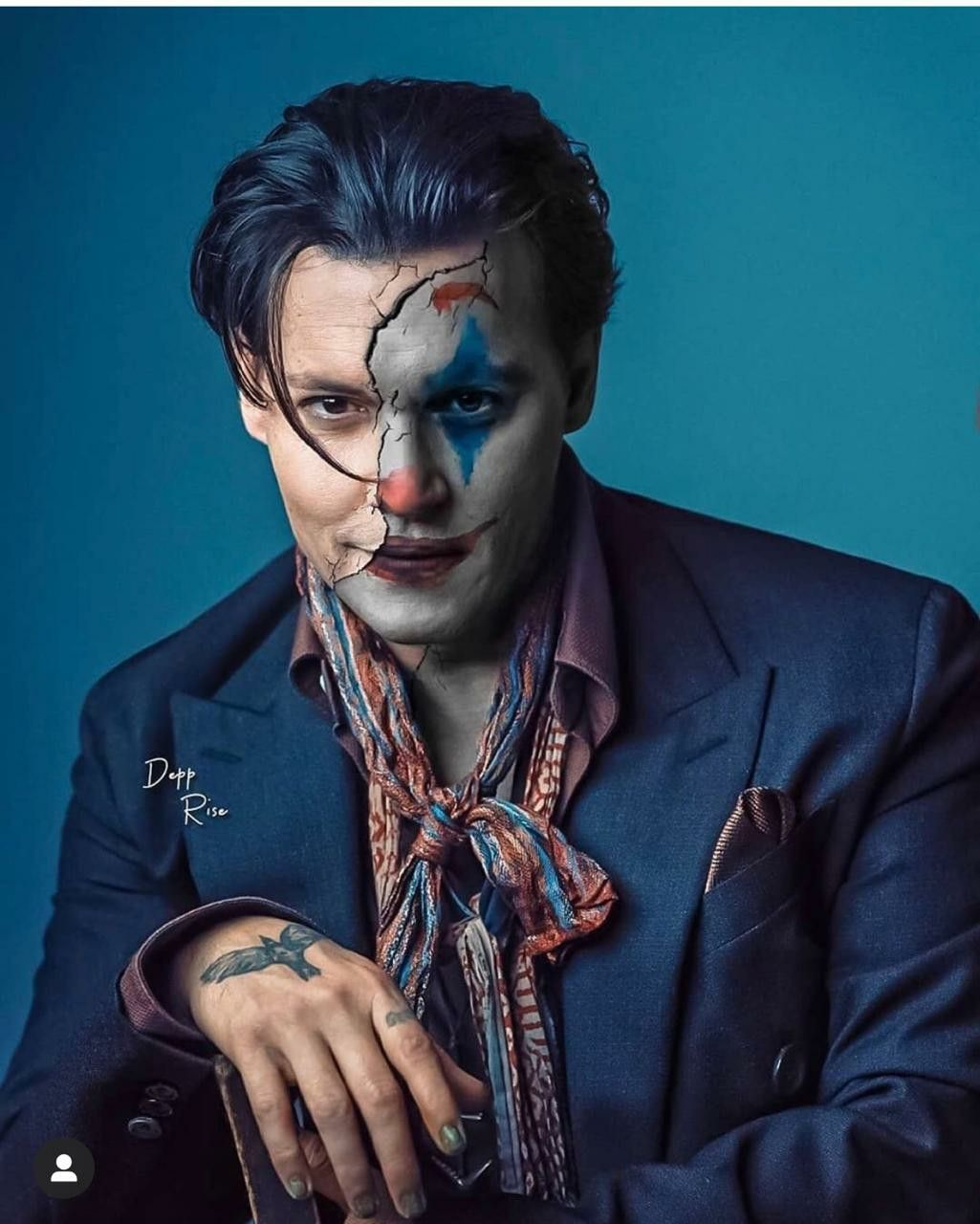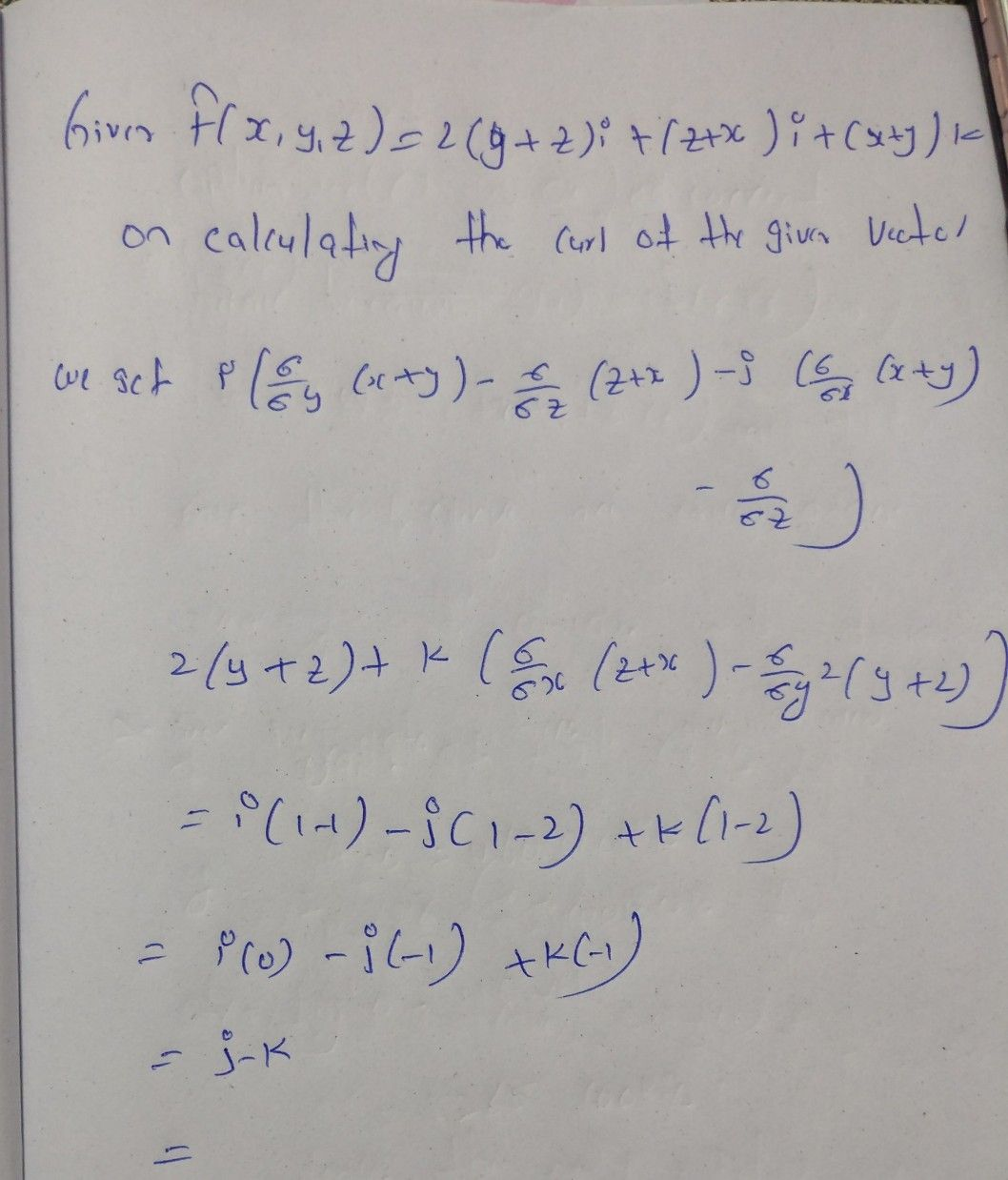Symbol
ProblemLet $F\left(x,y,z\right)=2\left(y+z\right)\vec{i} +\left(z+x\right)\vec{y} +\left(x+y\right)\bar{k}$ then curl $\vec{F} \left(x,y,z\right)$ $=a\right)\vec{0} b\right)$ $-\vec{j} c\right)$ $\vec{j} +\vec{k} d\right)$ $\vec{i} -\vec{k}$
Geometry
Search count: 114
SolutionQanda teacher - johny